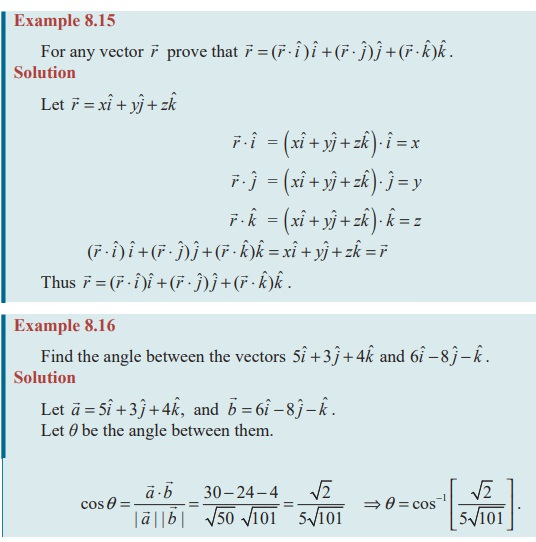Home | | Maths 11th std | Scalar product and Properties of Scalar Product

# Scalar product and Properties of Scalar Product

scalar product or dot product and Properties of Scalar Product

Scalar product

Definition 8.16

Letandbe any two non-zero vectors and θ be the included angle of the vectors as in Fig. 8.34.

Their scalar product or dot product is denoted byandis defined as a scalar |.| || cosθ .

Thus= || || cosθ .

Since the resultant ofis a scalar, it is called scalar product. Further we use the symbol dot (‘.’) and hence another name dot product.

## Geometrical meaning of scalar product (projection of one vector on another vector)

Let=,=and θ be the angle betweenand.

Draw BL perpendicular to OA. From the right triangle OLB

cosθ = OL/OBProperties of Scalar Product

(i) Scalar product of two vectors is commutative.

With usual definition,= || ||cosθ = || ||cosθ =That is, for any two vectorsand b ,=.

(ii)  Nature of scalar product

We know that 0 ≤ θ ≤ π .ProofNote 8.5

Suppose three sides are given in vector form, prove

(i) either sum of the vectors isor sum of any two vectors is equal to the third vector, to form a triangle.

(ii) dot product between any two vectors is 0 to ensure one angle is p/2 .

EXERCISE 8.3Study Material, Lecturing Notes, Assignment, Reference, Wiki description explanation, brief detail
11th Mathematics : UNIT 8 : Vector Algebra I : Scalar product and Properties of Scalar Product |